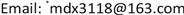1. 研究目的与研究内容

2. 模型1的建立：模糊层次分析法——模糊综合评价模型2.1. 模型背景

2.2. 模糊层次分析法的建立

1) 建立优先关系矩阵，既模糊互补矩阵 F = ( f i j ) n × n 。每一层次重的因素针对上层因素的相对重要性建立矩阵，一般由专家打分。这种矩阵是模糊互补矩阵。矩阵中的值用0.1~0.9标度确定(如表1所示)，这种标度计算简单，单相比较的两个元素之间的重要程度表现很模糊。为了能够准确的描述任意两个因素关于某准则的相对重要程度，这里采用如表1所示的0.1~0.9标度给予数量标度。

0.1~0.9 scale tabl

0.5同等重要两元素相比较，同等重要
0.6稍微重要两元素相比较，一元素比另一元素稍微重要
0.7明显重要两元素相比较，一元素比另一元素明显重要
0.8重要得多两元素相比较，一元素比另一元素重要得多
0.9极端重要两元素相比较，一元素比另一元素极端重要
0.1, 0.2, 0.3, 0.4反比较若元素 a i 与元素 a j 相比较得到判断 r i j ，则元素 a j 与元素 a i 相比较得到判断 r j i = 1 − r i j

2) 将优先关系矩阵改造成模糊一致矩阵 K n × n

k i j = k i − k j 2 n + 0.5

3) 计算排序向量 W 0

W ( 0 ) = [ ∑ j = 1 n r 1 j ∑ i = 1 n ∑ j = 1 n r i j , ∑ j = 1 n r 2 j ∑ i = 1 n ∑ j = 1 n r i j , ⋯ , ∑ j = 1 n r n j ∑ i = 1 n ∑ j = 1 n r i j ] T

4) 利用幂法求精度更高的排序向量A

‖ Y ( k + 1 ) ‖ − ‖ Y ( k ) ‖ < ε

ε 为给定误差，则 ‖ Y ( k + 1 ) ‖ 即为最大特征值

A = [ V k + 1 , 1 / ∑ i = 1 n V k + 1 , i , ⋯ , V k + 1 , n / ∑ i = 1 n V k + 1 , j ] T

2.3. 模糊评价模型

1) 根据评价目的确定评价指标集合

U = { u 1 , u 2 , ⋯ , u m }

2) 确定各评价指标的权重，这个指标就是FAHP中所确定的权重

w = { μ 1 , μ 2 , ⋯ , μ m }

∑ μ i = 1

3) 确定评价矩阵R

R = ( r i j )

4) 单因素模糊评价

S = w ∘ R = ( μ 1 , μ 2 , ⋯ , μ m ) ∘ ( r 11 r 12 ⋯ r 1 n r 21 r 22 ⋯ r 2 n ⋮ ⋮ ⋱ ⋮ r m 1 r m 2 ⋯ r m n ) = ( s 1 , s 2 , ⋯ , s n )

s k = ∨ j = 1 m ( μ j ∧ r j k ) = max 1 ≤ j ≤ m { min ( μ j , r j k ) } ,   k = 1 , 2 , ⋯ , n

s k = ∨ j = 1 m ( μ j ⋅ r j k ) = max 1 ≤ j ≤ m { μ j ⋅ r j k } ,   k = 1 , 2 , ⋯ , n

“ ⊕ ”是有界和运算，即在有界限制下的普通加法运算．对t个实数 x 1 , x 2 , ⋯ , x t 有

x 1 ⊕ x 2 ⊕ ⋯ ⊕ x t = min { 1 , ∑ i = 1 t x i }

s k = min { 1 , ∑ j = 1 m min ( μ j , r j k ) } ,   k = 1 , 2 , ⋯ , n

s k = ∑ j = 1 m ( a i ⋅ r i j ) ,   k = 1 , 2 , ⋯ , n

Fuzzy operator

M ( ∧ , ∨ )M ( ⋅ , ∨ )M ( ∧ , ⊕ )M ( + , ⋅ )

5) 得出综合结论：最大隶属原则

6) 多级模糊综合评判

U = { u 1 , u 2 , ⋯ , u m }

W = { μ 1 , μ 2 , ⋯ , μ m }

U i = { u i 1 , u i 2 , ⋯ , u i k } ， i = 1 , 2 , ⋯ , m

W i = { μ i 1 , μ i 2 , ⋯ , μ i k }

R i = [ r l j ] k × n , l = 1 , 2 , ⋯ , k

B = W ∘ ( W 1 ∘ R 1 W 2 ∘ R 2 ⋮ W m ∘ R m )

2.4. 评价模型的求解2.4.1. FAHP层次的构建

Construction of FAHP leve

2.4.2. FAHP指标权重的计算

Voting result

(A)商家 (B1)99食品价格 (C11)9

F i j = { 0.5 + 0.5 e       当 n ( i ) > n ( j ) 0.5 − 0.5 e       当 n ( i ) ≤ n (j)

2.4.3. FSEM部分的求解

S = w ∘ R = ( μ 1 , μ 2 , ⋯ , μ m ) ∘ ( r 11 r 12 ⋯ r 1 n r 21 r 22 ⋯ r 2 n ⋮ ⋮ ⋱ ⋮ r m 1 r m 2 ⋯ r m n ) = ( s 1 , s 2 , ⋯ , s n )

Evaluation matrix and index weigh

[ A B1 B2 B3 B1 0.5000 0.9091 0.6010 B2 0.0909 0.5000 0.6235 B3 0.3990 0.3765 0.5000 ][0.45720.26570.2771]
[ B1 C11 C12 C13 C14 C11 0.5000 0.4591 0.4262 0.2750 C12 0.5409 0.5000 0.7773 0.5909 C13 0.5738 0.2227 0.5000 0.6639 C14 0.7250 0.4091 0.3361 0.5000 ][0.20880.30440.24280.2440]
[ B2 C21 C22 C23 C24 C21 0.5000 0.1939 0.6000 0.7500 C22 0.8061 0.5000 0.5612 0.6531 C23 0.4000 0.4388 0.5000 0.3000 C24 0.2500 0.3469 0.7000 0.5000 ][0.25180.32060.20520.2224]
[ B3 C31 C32 C33 C34 C31 0.5000 0.3500 0.4000 0.4318 C32 0.6500 0.5000 0.1667 0.2727 C33 0.6000 0.8333 0.5000 0.1591 C34 0.5682 0.7273 0.8409 0.5000 ][0.20790.19830.25590.3379]

Weight tabl

(A)商家 (B1)0.4572食品价格 (C11)0.2088

Evaluation form of respondent

First level fuzzy evaluation of Meituan takeou

B20.1566440.1833160.206110.2643070.189622
B30.245190.3236940.2299280.1532860.047902

B20.1065530.1850660.2070620.3234310.177887
B30.2722310.3234530.2156350.1437570.044924

B20.1251770.1840690.2578780.2629930.169883
B30.2664290.3126640.2614480.1281920.031266

B20.0977920.1930890.2590070.2960090.154103
B30.3160950.3016590.2438340.1111240.027288

Final evaluation results of Meituan takeou

2.5. 模型的优缺点

1) FAHP-FSEM模型有机结合了模糊层次分析法和模糊综合评价法，既将诸多不便于量化的因素以权重，评价矩阵的形式有效表示，又克服了一般AHP方法难以构建合乎要求的矩阵的问题。

2) 在FAHP-FSEM模型中，FAHP仅用于求出各级指标的权重，在求出总指标的过程中，不再使用AHP的多级权重相乘。

3) 模型能做到因人而异，消费者可以根据自身的喜好在FAHP模型中设定权重，然后根据FSEM中根据与自己环境，喜好相似的群体评价，得出适合于自己的外卖平台排序。同理，对于商家，也可以根据对周围环境的调研，对商家自身的改进有很大的参考意义。

1) 在FAHP模型中，考虑到的因素及其仍可以增多，可以设定更高一级的层次，使得最终结果更有说服力。

2) 在FSEM模型中，我们使用了最大隶属原则来评定不同平台的评价在不同算子下对应的星级，实际上，最大隶属原则由于只考虑了概率最大的星级，而过滤去了很多信息。在今后的进一步研究中，可以采用平均值法等其他原则。

3. 关于外卖平台送餐的优化问题讨论3.1. 问题背景和研究思路

3.2. 建模讨论

1) 客户取餐时间控制在较小的区间：如果只设立一个临时外卖柜，外卖员的工作量的确可以大大减小，但是客户取餐的时间上升将会导致对外卖的需求量大幅下降。

2) 将成本控制于合理的区间：如果在区域内设立过多的临时外卖柜，则加重了外卖员的负担，在经济上也是不合理的。但是在实际的商业运作中，在外卖需求旺盛的区域，尤其是核心商圈的每一栋大楼下都设定一个外卖柜是可行的。

2019年高等学校大学数学教学研究与发展中心教学改革项目(CMC20190412)；中国石油大学(北京)教学改革项目(21G16016 & 29G17006)。